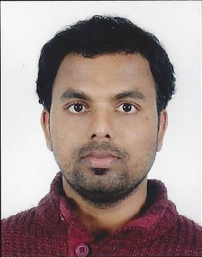# Integral and Vector Calculus

By Prof. Hari Shankar Mahato   |   IIT Kharagpur
Learners enrolled: 1317
This course will offer a detailed introduction to integral and vector calculus. We’ll start with the concepts of partition, Riemann sum and Riemann Integrable functions and their properties. We then move to anti-derivatives and will look in to few classical theorems of integral calculus such as fundamental theorem of integral calculus. We’ll then study improper integral, their convergence and learn about few tests which confirm the convergence. Afterwards we’ll look into multiple integrals, Beta and Gamma functions, Differentiation under the integral sign. Finally, we’ll finish the integral calculus part with the calculation of area, rectification, volume and surface integrals. In the next part, we’ll study the vector calculus. We’ll start the first lecture by the collection of vector algebra results. In the following weeks, we’ll learn about scalar and vector fields, level surfaces, limit, continuity, and differentiability, directional derivative, gradient, divergence and curl of vector functions and their geometrical interpretation. We’ll also study the concepts of conservative, irrotational and solenoidal vector fields. We’ll look into the concepts of tangent, normal and binormal and then derive the Serret-Frenet formula. Then we’ll look into the line, volume and surface integrals and finally we’ll learn the three major theorems of vector calculus: Green’s, Gauss’s and Stoke’s theorem.

INTENDED AUDIENCE : Mathematics
PREREQUISITES : Differential calculus of one and several variables.
INDUSTRY SUPPORT : None
Summary
 Course Status : Completed Course Type : Core Duration : 12 weeks Start Date : 24 Jan 2022 End Date : 15 Apr 2022 Exam Date : 23 Apr 2022 IST Enrollment Ends : 07 Feb 2022 Category : Mathematics Credit Points : 3 Level : Undergraduate

### Course layout

Week 1  :  Partition, concept of Riemann integral, properties of Riemann integrable functions, anti-derivatives, Fundamental theorem of Integral calculus, mean value theorems.
Week 2  :  Reduction formula and derivation of different types of formula, improper integrals and their convergence, tests of convergence.
Week 3  :  Beta and Gamma function, their properties, differentiation under the integral sign, Leibnitz rule.
Week 4  :  Double integrals. change of order of integration, Jacobian transformations, triple integrals.
Week 5  :  Area of plane regions, rectification, surface integrals.
Week 6  :  Volume integrals, center of gravity and moment of Inertia.
Week 7  :  Collection of vector algebra results, scalar and vector fields, level surfaces, limit, continuity, differentiability of vector functions
Week 8  :  Curves, Arc-length, partial derivative of vector function, directional derivative gradient, divergence and curl.
Week 9  :  Irrotational, conservative and Solenoidal fields, tangent, normal, binormal, Serret-Frenet formula.
Week 10  :  Application of vector calculus in mechanics, lines, surface and volume integrals. line integrals independent of path.
Week 11  :  The divergence theorem of Gauss, Stokes theorem, and Green’s theorem.
Week 12  :  Integral definition of gradient, divergence and curl. revision of problems from Integral and Vector calculus.

### Books and references

Differential and Integral Calculus by N. Piskunov.2. Integral Calculus by Shanti Narayan and P.K. Mittal.3. Principles of Mathematical Analysis by W. Rudin4. Real Analysis by S.K. Mapa5. Advanced Engineering Mathematics, 9th edition by E. Kreyszig6. Vector Analysis by Ghosh and Maity7. An Introduction to Vector Analysis: For Physicists and Engineers by B. Hague8. Vector Analysis by H.E.Newell, Jr.

### Instructor bio### Prof. Hari Shankar Mahato

IIT Kharagpur
Prof. Hari Shankar Mahato is currently working as an Assistant Professor in the Department of Mathematics at the Indian Institute of Technology Kharagpur. Before joining here, he worked as a postdoc at the University of Georgia, USA. He did his PhD from the University of Bremen, Germany and then he worked as a Postdoc at the University of Erlangen-Nuremberg and afterwards at the Technical University of Dortmund, both located in Germany. His research expertise are Partial Differential Equations, Applied Analysis, Variational Methods, Homogenization Theory and very recently he has started working on Mathematical Biology. He can be able to teach (both online and offline) any undergraduate courses from pre to advanced calculus, mechanics, ordinary differential equations, up to advanced graduate courses like linear and nonlinear PDEs, functional analysis, topology, mathematical modeling, fluid mechanics and homogenization theory

### Course certificate

The course is free to enroll and learn from. But if you want a certificate, you have to register and write the proctored exam conducted by us in person at any of the designated exam centres.
The exam is optional for a fee of Rs 1000/- (Rupees one thousand only).
Date and Time of Exams: 23 April 2022 Morning session 9am to 12 noon; Afternoon Session 2pm to 5pm.
Registration url: Announcements will be made when the registration form is open for registrations.
The online registration form has to be filled and the certification exam fee needs to be paid. More details will be made available when the exam registration form is published. If there are any changes, it will be mentioned then.
Please check the form for more details on the cities where the exams will be held, the conditions you agree to when you fill the form etc.

CRITERIA TO GET A CERTIFICATE

Average assignment score = 25% of average of best 8 assignments out of the total 12 assignments given in the course.
Exam score = 75% of the proctored certification exam score out of 100

Final score = Average assignment score + Exam score

YOU WILL BE ELIGIBLE FOR A CERTIFICATE ONLY IF AVERAGE ASSIGNMENT SCORE >=10/25 AND EXAM SCORE >= 30/75. If one of the 2 criteria is not met, you will not get the certificate even if the Final score >= 40/100.

Certificate will have your name, photograph and the score in the final exam with the breakup.It will have the logos of NPTEL and IIT Kharagpur .It will be e-verifiable at nptel.ac.in/noc.

Only the e-certificate will be made available. Hard copies will not be dispatched.

Once again, thanks for your interest in our online courses and certification. Happy learning.

- NPTEL team